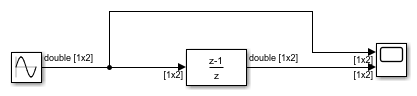Documentation

## Difference Sine Wave Signal

This example shows how to calculate the difference in a sine wave signal at each time step. The input is a 1-by-2 vector of sine waves, with amplitude 1 and 3. The difference block calculates the difference in each sine wave signal at every time step. The Scope block displays both the original sine waves and the output of the difference block.